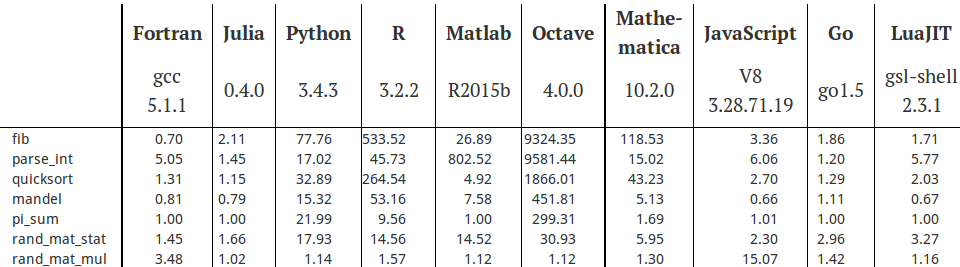# 随机数生成

Matlab通过内置的rng函数指定不同的发生器，其中包括1995年Matlab采用George Marsaglia 在1991年提出的借位减（subtract with borrow，简称SWB）发生器。在Matlab中，设置如下命令可指定发生器及其状态，其中1234是随机数种子，指定发生器的状态，目的是重复实验结果，v5uniform是发生器的名字。

rng(1234, 'v5uniform')


Octave通过内置的rand函数指定发生器的不同状态，为获取相同的两组随机数，state参数得设置一样，如1234（你也可以设置为别的值）。Octave已经放弃了老版本内置的发生器，找不到命令去指定早期的发生器，这个和Matlab不一样。

rand ('state',1234)
rand(1,5)

   0.9664535   0.4407326   0.0074915   0.9109760   0.9392690

rand ('state',1234)
rand(1,5)

   0.9664535   0.4407326   0.0074915   0.9109760   0.9392690


Python的numpy模块也采用MT发生器，类似地，通过如下方式获得相同的两组随机数

import numpy as np
a = np.random.RandomState(1234)
a.rand(5)
array([ 0.19151945,  0.62210877,  0.43772774,  0.78535858,  0.77997581])

a = np.random.RandomState(1234)
a.rand(5)
array([ 0.19151945,  0.62210877,  0.43772774,  0.78535858,  0.77997581])


R的默认发生器也是MT发生器，除了设置随机数种子的seed参数，还可以通过kind参数指定其他发生器，normal.kind参数指定产生正态分布随机数的发生器，下面也使用类似的方式产生两组完全一样的随机数。

set.seed(seed, kind = NULL, normal.kind = NULL)
set.seed(1234,kind = "Mersenne-Twister", normal.kind =  "Inversion") # 默认参数设置
runif(5)
 0.1137034 0.6222994 0.6092747 0.6233794 0.8609154

set.seed(1234,kind = "Mersenne-Twister", normal.kind =  "Inversion") # 默认参数设置
runif(5)
 0.1137034 0.6222994 0.6092747 0.6233794 0.8609154


SWB发生器中“借位相减”步骤是指序列的第$i$个随机数$z_{i}$要依据如下递推关系产生，$$z_{i}=z_{i+20}-z_{i+5}-b$$ 下标$i,i+20,i+5$都是对32取模的结果，$z_{i+20}$$z_{i+5}$做减法运算，$b$是借位，其取值与前一步有关，当$z_i$是正值时，下一步将$b$置为0，如果计算的$z_i$是负值，在保存$z_i$之前，将其值加1，并在下一步，将$b$的值设为$2^{-53}$

Matlab内置的发生器及大部分的函数，底层实现都是C或者Fortran，MathWorks创始人Cleve B. Moler是数值分析领域著名的LINPACK和EISPACK包的作者之一，他当年做了很多优化和封装，进而推出Matlab，只要是调用内置函数，效率不会比C差，自己写的含有循环、判断等语句的代码，性能就因人而异了，对大多数人来说，性能要比C低。这里比较Matlab内置SWB发生器（就当作是C语言实现的好了）和用Matlab重写的SWB发生器的效率，代码如下：

% matlab code
tic % 大约几秒
rng(1234, 'v5uniform') % 调用SWB发生器
x = rand(1,2^24);
toc

% octave code
id = tic % 时间耗费大约一小时
randtx('state',0)
x = randtx(1,2^24);
toc (id)


randtx不是Matlab和Octave内置的函数，而是Cleve B. Moler基于Matlab实现的SWB发生器，也是100多行包含嵌套循环等语句的Matlab代码打包的函数，上面的代码运行时间差异之大也就不难理解了，为了能在Octave上跑，我做了少量修改，Octave软件版本为4.2.1，安装Octave时，Blas选择OpenBlas，为了后续检验，在获得随机数后，将其保存到磁盘文件 random_number.mat

save -mat random_number.mat x


R，Octave，Matlab和Python内置的发生器都是MT发生器，与之实现有关的数学库，也是Blas，虽然有开源和进一步优化的商业版本之分，但是矩阵乘法，向量乘法之类运算的效率也就半斤八两，Julia语言官网给出了一个标准测试2# 统计检验

## 相关性检验

library(ggplot2)
set.seed(1234)
corr <- rep(0, 1000)
for(i in seq(from = 1000, to = 1000000, by = 1000)) {
corr[i/1000] <-  cor.test(runif(i, min = 0, max = 1),
runif(i, min = 0, max = 1))$estimate} ggplot(data.frame(x = seq(1000), y = corr), aes(x = x, y = y)) + geom_hex(show.legend = FALSE) + scale_fill_viridis_c(direction = -1) + xlab("Sample size *10^3") + ylab("Correlation")## 分布检验 检验产生的随机数是否服从指定的分布：原假设是样本来自指定的分布，计算的P值比较大，就不能拒绝原假设。 ks.test(runif(1000), "punif") # 分布检验 ## ## One-sample Kolmogorov-Smirnov test ## ## data: runif(1000) ## D = 0.022302, p-value = 0.7025 ## alternative hypothesis: two-sided  检验两样本是否来自同一分布：原假设是两样本来自同一分布，计算的P值比较小，就表示两样本不是来自同一分布。 ks.test(runif(1000), runif(1000)) # 同分布检验 ## ## Two-sample Kolmogorov-Smirnov test ## ## data: runif(1000) and runif(1000) ## D = 0.04, p-value = 0.4005 ## alternative hypothesis: two-sided  从结果来看，R内置的随机数发生器通过了检验（嘿嘿，这是肯定的！！）。 ## 游程检验 游程检验对随机数序列的随机性检验，不对序列做任何分布假设，不同于上面的相关性检验和省略没讲的特征统计量（如均值和方差）的检验。先对随机性略作解释，简单起见，我们考虑0-1序列，抛掷均匀的硬币1000次，正面向上记为1，反面向上记为0，这是一个离散的均匀分布，每一次抛掷硬币都无法准确地判断出现的是正面还是反面，若记录的序列中0和1相对集中的出现，不是随机，0和1交替出现，呈现周期性也不是随机，除了这两种情况基本就是随机了。 游程检验的原假设是序列满足随机性，序列一旦生成，就是有序的，因为游程检验需要固定位置，再数上升（下降）的游程数，当计算的P值比较大时，不能拒绝原假设，即不能否认这个序列是随机的。对上述0-1序列进行模拟，然后检验，如下所示 library(tseries) x <- sample(c(0, 1), 1000, replace = TRUE, prob = c(1/2, 1/2)) runs.test(factor(x)) ## ## Runs Test ## ## data: factor(x) ## Standard Normal = 0.45116, p-value = 0.6519 ## alternative hypothesis: two.sided  现在用游程检验比较SWB发生器（Octave/Matlab版）、MT发生器（R语言版）和MT发生器（C语言版）。对于一般的实数序列，得先指定一个阈值，记为delta，然后比较序列中的值和delta的大小关系，这里类似数上升或下降的游程长度（runs length），基于这样一个事实：如果序列表现随机，则序列中两个小于delta的值，间隔越远出现的次数越少。可以这样理解，还是以上面抛硬币的例子来说，出现了很多次正面，那么下一次抛掷倾向于出现反面，这是一条人人可接受的经验。 为了把这条经验可视化出来，对序列做如下操作：先给定阈值delta为0.01（也可以取别的值），取出序列中的值小于delta的位置，位置序列前面添加0，再差分，然后每个值减1，得到新序列，新序列中的值为0，表明原序列连续两个值小于delta，新序列中的值为1，表明间隔1个数小于delta，新序列中的值为2，表明间隔2个数小于delta，依次类推…..统计所有的情况，用直方图显示，这就获得游程长度与间隔的关系图（间隔数取到100足可示意）。 library(gridExtra) library(R.matlab) # 游程频数直方图 run_test_fun <- function(x, string, delta) { n <- length(x) len <- diff(c(0, which(x < delta), n + 1)) - 1 ggplot(data.frame(x = len[len < 101]), aes(x, fill = ..count..)) + scale_fill_viridis(direction = -1) + geom_histogram(binwidth = 1, show.legend = FALSE) + xlab(string) + ylab("") } set.seed(1234) # R默认采用Mersenne Twister发生器 r_data <- runif(2^24, 0, 1); # R内生成均匀分布随机数 matlabv5_data <- readMat("random_number.mat") # 读取Octave生成的均匀分布随机数 temp <- read.table(file = "random_number.txt") # 读取C语言生成的均匀分布随机数 c_data <- c(as.matrix(t(temp))) p1 <- run_test_fun(x = r_data, string = "R", delta = 0.01) p2 <- run_test_fun(x = matlabv5_data$x, string = "Matlab v5", delta = 0.01)
p3 <- run_test_fun(x = c_data, string = "C", delta = 0.01)
grid.arrange(p1, p2, p3, ncol=3)# 应用

## 两个均匀分布的统计模拟

set.seed(1234)
x <- runif(10000, min = 0, max = 1)
y <- runif(10000, min = 0, max = 1)
z <- x + y
plot(z) # 散点图
hist(z) # 直方图


ggplot(data.frame(x = seq(10000), y = z), aes(x = x, y = y)) +
geom_hex(show.legend = FALSE) +
scale_fill_viridis_c(direction = -1) + xlab("") + ylab("")p4 <- ggplot(data.frame(x = z), aes(x, fill = ..count..)) +
scale_fill_viridis_c(direction = -1) +
geom_histogram(bins=20, show.legend = FALSE) + xlab("") + ylab("")
p5 <- ggplot(data.frame(x = sqrt(6)*(z-1)), aes(x, fill = ..count..)) +
scale_fill_viridis_c(direction = -1) +
geom_histogram(bins = 20, show.legend = FALSE) + xlab("") + ylab("")
grid.arrange(p4, p5, ncol=2)ks.test(sqrt(6)*(z-1), "pnorm") # 分布检验
##
## One-sample Kolmogorov-Smirnov test
##
## data: sqrt(6) * (z - 1)
## D = 0.025778, p-value = 3.381e-06
## alternative hypothesis: two-sided


## 精确分布的推导及计算

$$\int_{-\infty}^{+\infty}\vert \varphi_2(t) \vert \mathrm{d} t < \infty$$

$S_2$的密度函数就可以表示为

$$p_2(x)=\frac{1}{2 \pi}\int_{-\infty}^{+\infty}\mathrm{e}^{-itx}\varphi_2(t)\mathrm{d}t$$

$$\varphi_n(t)=\big(\frac{e^{it}-1}{it}\big)^n=\big(\frac{\sin(t/2)\mathrm{e}^{\frac{it}{2}}}{t/2}\big)^n$$ 那么，同理 $$p_n(x)=\frac{2}{\pi}\int_{0}^{+\infty}\cos\big(2(n/2-x)t\big)(\frac{\sin(t)}{t})^n\mathrm{d}t$$

integrate(function(t,x,n) 2/pi*cos((n-2*x)*t)*(sin(t)/t)^n ,x = 1,n = 2,
lower = 0,upper = Inf,subdivisions = 1000)
## 0.9999846 with absolute error < 6.6e-05


$$p_2(x)=\frac{1}{2}\big((2-x)\mathrm{sgn}(2-x)-x\mathrm{sgn}(-x)\big)-(1-x)\mathrm{sgn}(1-x)=\frac{1}{2}(\left | x \right |+\left | x-2 \right |)-\left | x-1 \right |,0<x<2$$

$p_2(x)$的密度函数图象如下：

fun_p2_1 <- function(x) { 1 / 2 * (abs(x - 2) - 2 * abs(x - 1) + abs(x)) }
fun_p2_2 <- function(x) {
x <- as.matrix(x)
tempfun <- function(x) {
integrate(function(t, x, n) 2 / pi * cos((n - 2 * x) * t) * (sin(t) / t) ^ n,